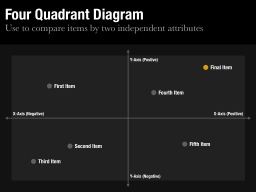Use these Quadrant Diagrams slides to present a diagram that compares items by two independent, continuous attributes. There are two slides available in this slide set: 1) a large Quadrant Diagram that fills the slide and 2) a smaller Quadrant Diagram that provides an bullet point area for an explanation.

## What is a Quadrant Diagram?

The Quadrant Diagram is a variation of the 2x2 Matrix. If you haven't already, please review the 2x2 Matrix page which provides more background on how to use this slide.

### The Quadrant Diagram vs. The 2x2 Matrix

Slidevana contains two similar slide sets: the 2x2 Matrix and the Quadrant Diagram. Both types of slides are used to compare items using two independent attributes. There are two primary differences between the slides:

• Discrete vs. Continuous. In general, the 2x2 Matrix is used to categorize items into four discrete buckets. This means that the attributes of a 2x2 Matrix have discrete values. For example, if Growth Rate is an attribute in a 2x2 Matrix, the value of Growth Rate for any item can either be "Low" or "High", but not somewhere in between. Conversely, the attributes of a Quadrant Diagram have continuous values. An item can fall anywhere on the continuum from the attribute's lowest value to its highest value.

• Point of Origin. Unlike the 2x2 Matrix, the Quadrant Diagram has a center point, or point of origin, which may be meaningful for the data plotted on the Quadrant Diagram. Depending on the axes (attributes) of the Quadrant Diagram, this center point may signify the changeover from negative to positive or may represent the point at which the two opposites of an attribute are in balance.

## Tips for Using the Quadrant Diagram

The Quadrant Diagram is one of those rare frameworks which is both simple to understand and powerful to use. Here are some tips to get the most out of the Quadrant Diagram:

### 1. Select Independent Attributes

Like the 2x2 Matrix, a Quadrant Diagram is most effective when plotting two attributes that are independent. For more information on independent attributes, refer to the 2x2 Matrix page.

Unlike the 2x2 Matrix, the two attributes are represented by an x-axis and a y-axis, and an item can take on any value on the continuum from that attribute's low point to its high point. Rather than categorizing items into four discrete buckets (as you would with a 2x2 Matrix), you plot items on the Quadrant Diagram in the same way that you would plot points on graph paper.

### 3. Identify Key Items

The Quadrant Diagram is often used to show how one or more key items compares to the rest of the items in the set. These key items are often in the upper right quadrant of the Quadrant Diagram and/or are outliers from the rest of the set. In order to draw attention to these key items, you should highlight them with a bold color so that they stand out to your audience. This makes it easier for your audience to understand and process the message of your slide.All PowerPoint Templates (includes Four Quadrant Diagram PowerPoint Templates plus all other slides) \$79All Keynote Templates (includes Four Quadrant Diagram Keynote Templates plus all other slides) \$79Both PowerPoint and Keynote Templates (includes Four Quadrant Diagram PowerPoint and Keynote Templates plus all other slides) \$99